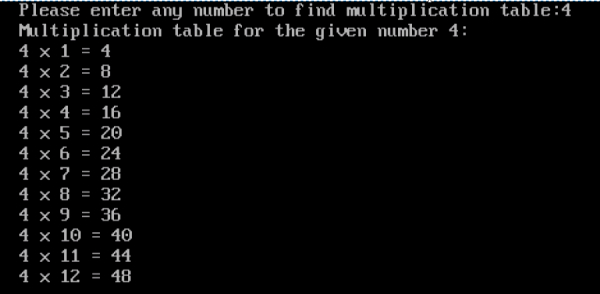# Print multiplication table of a given number in C

## Program Description

Print multiplication table of a given number

## Algorithm

Accept any number from the User for which we need to form multiplication table.

Multiply the given number starting with the value of I (=1)

Multiply the given number by incrementing the value of I till the I value is lesser than or equal to 12.

## Example

/* Program to print the multiplication table of a given number */
#include <stdio.h>
int main() {
int number, i;
clrscr();
printf("Please enter any number to find multiplication table:");
scanf("%d", &number);
printf("Multiplication table for the given number %d: ", number);
printf("");
for(i=1;i<=12;i++){
printf("%d x %d = %d", number, i, number * i);
printf("");
}
getch();
return 0;
}

## Output